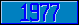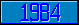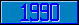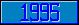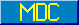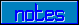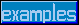## \$%CRC16^STRING

### M[UMPS] by Example

Approved for inclusion in a future M[UMPS] language standard as part of the library for cyclic redundancy checks.

This function computes a Cyclic Redundancy Code of the 8-bit character string string, using X16 + X15 + X2 + 1 as the polynomial. The optional parameter seed may supply an initial value, which allows for running CRC calculations on multiple strings. If the parameter seed is not specified, a default value of 0 is assumed. The value of seed is limited to 0 ≤ seed < 216. The function value will be between 0 and 216.

A typical method of transmitting data is to send data followed by a check-sum value, e.g.:

```

Set CRC=\$Extract(Data,L–1,L)

Set VAL=0

For i=1:1:2 Set VAL=VAL*256+\$ASCII(CRC,i)

Set DATA=\$Extract(Data,1,L–2)

If \$%CRC16^STRING(Data)'=VAL Do Error

```

A check-sum can also be calculated over multiple strings:

```
Set(I,C)=0

For  Set I=\$Order(X(I)) Quit:'I  Do

. Set C=\$%CRC16^STRING(X(I),C)

```
Reference Value \$%CRC16^STRING(123) 47620 \$%CRC16^STRING(1234) 5306 \$%CRC16^STRING(12345) 42069 \$%CRC16^STRING(" ") 55297 \$%CRC16^STRING("1234567890123456789012345678901234567890") 57484 \$%CRC16^STRING("ABCDEFGHIJKLMNOPQRSTUVWXYZ") 6375 \$%CRC16^STRING("a") 59585 \$%CRC16^STRING("abc") 38712 \$%CRC16^STRING("abcd") 14743 \$%CRC16^STRING("abcdef") 22533 \$%CRC16^STRING("abcdefghijklmnopqrstuvwxyz") 39965

The MDC has approved code to approximate the return value of this function. Implementors are encouraged to provide more accurate and efficient code).